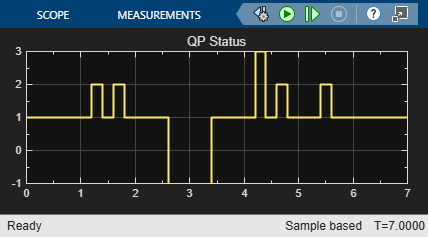Documentation

## Monitoring Optimization Status to Detect Controller Failures

This example shows how to use the `qp.status` outport of the MPC Controller block in Simulink® to detect controller failures in real time.

### Overview of Run-Time Control Monitoring

The `qp.status` output from the MPC Controller block returns a positive integer when the controller finds an optimal control action by solving a quadratic programming (QP) problem. The integer value corresponds to the number of iterations used during optimization. If the QP problem formulated at a given sample interval is infeasible, the controller will fail to find a solution. In that case, the MV outport of controller block retains the most recent value and the `qp.status` outport returns -1. In a rare case when the maximum number of iteration is reached during optimization, the `qp.status` outport returns 0.

In industrial MPC applications, you can detect whether your model predictive controller is in a failure mode (0 or -1) or not by monitoring the `qp.status` outport. If an MPC failure occurs, you can use this signal to switch to a backup control plan.

This example shows how to setup run-time controller status monitoring in Simulink.

### Define Plant Model

The test plant is a single-input, single-output plant with hard limits on both manipulated variable and controlled output. A load disturbance is added at the plant output. The disturbance consists of a ramp signal that saturates manipulated variable due to the hard limit on the MV. After saturation occurs, you lose the control degree of freedom and the disturbance eventually forces the output outside its upper limit. When that happens, the QP problem formulated by the model predictive controller at run-time becomes infeasible.

Define the plant model as a simple SISO system with unity gain.

```Plant = tf(1,[2 1]); ```

Define the unmeasured load disturbance. The signal ramps up from 0 to 2 between 1 and 3 seconds, then ramps down from 2 to 0 between 3 and 5 seconds.

```LoadDist = [0 0; 1 0; 3 2; 5 0; 7 0]; ```

### Design MPC Controller

Create MPC object.

```Ts = 0.2; Obj = mpc(Plant, Ts); ```
```-->The "PredictionHorizon" property of "mpc" object is empty. Trying PredictionHorizon = 10. -->The "ControlHorizon" property of the "mpc" object is empty. Assuming 2. -->The "Weights.ManipulatedVariables" property of "mpc" object is empty. Assuming default 0.00000. -->The "Weights.ManipulatedVariablesRate" property of "mpc" object is empty. Assuming default 0.10000. -->The "Weights.OutputVariables" property of "mpc" object is empty. Assuming default 1.00000. ```

Define hard constraints on plant input (MV) and output (OV). By default, all the MV constraints are hard and OV constraints are soft.

```Obj.MV.Min = -1; Obj.MV.Max = 1; Obj.OV.Min = -1; Obj.OV.Max = 1; ```

Configure the upper and lower OV constraints as hard bounds.

```Obj.OV.MinECR = 0; Obj.OV.MaxECR = 0; ```

Override the default estimator. This high-gain estimator improves detection of an impending constraint violation.

```setEstimator(Obj,[],[0;1]) ```

To run this example, Simulink® is required.

```if ~mpcchecktoolboxinstalled('simulink') disp('Simulink(R) is required to run this example.') return end ```

Build the control system in a Simulink model and enable the `qp.status` outport from the controller block dialog. Its run-time value is displayed in a Simulink Scope block.

```mdl = 'mpc_onlinemonitoring'; open_system(mdl) ```Simulate the closed-loop response.

```open_system([mdl '/Controller Status']) open_system([mdl '/Response']) sim(mdl) ```
```-->Converting the "Model.Plant" property of "mpc" object to state-space. -->Converting model to discrete time. -->Assuming output disturbance added to measured output channel #1 is integrated white noise. -->The "Model.Noise" property of the "mpc" object is empty. Assuming white noise on each measured output channel. ```As shown in the response scope, the ramp-up disturbance signal causes the MV to saturate at its lower bound -1, which is the optimal solution for these situations. After the plant output exceeds the upper limit, at the next sampling interval (2.6 seconds), the controller realizes that it can no longer keep the output within bounds (because its MV is still saturated), so it signals controller failure due to an infeasible QP problem (-1 in the controller status scope). After the output comes back within bounds, the QP problem becomes feasible again (3.4 seconds). Once the MV is no longer saturated, normal control behavior returns.

```bdclose(mdl) ```